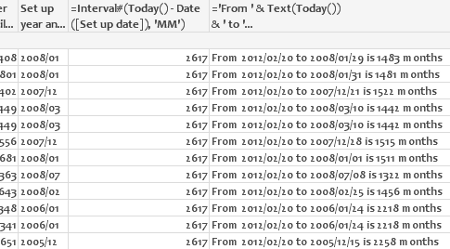QlikView Creating Analytics

Discussion Board for collaboration related to Creating Analytics for QlikView.Not applicable

How Do I Get the Number of Months Between Two Dates?

Other posts here in the forii have mentioned Interval() to get the number of days or months between two days.  However, I have not found a format code to get months.  Either of these expressions...

=Interval#(Today() - [Set up date], 'YY-MM-DD')

=Interval#(Today() - [Set up date], 'YY-MM')

...give me the number of days since [Set up date], but I need months.  Using 'MM' gives the value "2614" for all dates from 2005 to yesterday - I have no idea where that number comes from.  The doc page for Interval() is of course useless.

If there's a simpler way to get the number of months between two dates, such as is present in any RDMS (e.g., DATEDIFF() in MSSQL and MySQL, MONTHS_BETWEEN() in Oracle), I am all ears.  If someone suggests adding the difference in years times 12 to the difference in months, I fear I may lose any remaining faith in QlikView.

Thank you!

Tags (1)
1 Solution

Accepted Solutions
HighlightedMVP & Luminary

Re: How Do I Get the Number of Months Between Two Dates?

Sorry to shake your faith in QV, but the expression I would use is:

=((year(today(2))*12)+month(today(2))) - (((year([Set up date])*12)+month([Set up date])))

You can ease your pain somewhat by creating a variable function in the script:

SET MonthDiff=((year(today(2))*12)+month(today(2))) - (((year(\$1)*12)+month(\$1)));

and then in the chart:

=\$(MonthDiff([Set up date]))

-Rob

24 RepliesPartner

How Do I Get the Number of Months Between Two Dates?

You should be able to do the same formula in qlikview.

http://support.microsoft.com/kb/214134Not applicable

How Do I Get the Number of Months Between Two Dates?

You could always create your own custom function in the module:

Function MonthDiff (startdate, enddate)

' return number of months between two dates

If IsDate(startdate) and IsDate(enddate) Then

MonthDiff = DateDiff("m", startdate, enddate)

End If

End Function

then just use it in the script eg

MonthDiff(text(W1DEL1), text(today()))) * 12))

I never did find an easier way myself!

Regards,

Gordon

Esteemed Contributor III

How Do I Get the Number of Months Between Two Dates?

Jonathan,

Since "Month" is not a fixed length interval, you have to define a rule.  For practical purposes, this can be a reasonable approximation:

round((interval#(Today()-[Set up date], 'DD')/30.5), 0.1)

HighlightedMVP & Luminary

Re: How Do I Get the Number of Months Between Two Dates?

Sorry to shake your faith in QV, but the expression I would use is:

=((year(today(2))*12)+month(today(2))) - (((year([Set up date])*12)+month([Set up date])))

You can ease your pain somewhat by creating a variable function in the script:

SET MonthDiff=((year(today(2))*12)+month(today(2))) - (((year(\$1)*12)+month(\$1)));

and then in the chart:

=\$(MonthDiff([Set up date]))

-Rob

Esteemed Contributor

How Do I Get the Number of Months Between Two Dates?

ue below  code

Interval(Today() - [Set up date], 'mm')Not applicable

Re: How Do I Get the Number of Months Between Two Dates?

The EDate() and LDate() functions, useful though they are in Excel, do not exist in QlikView.Not applicable

Re: How Do I Get the Number of Months Between Two Dates?

I'm specifically looking for an expression in the QlikView document itself (e.g., a dimension in a pivot table), not the script.  There are over a dozen date metrics in the data set, so it would be impractical to calculate all possible date differences in the script.Not applicable

Re: How Do I Get the Number of Months Between Two Dates?

The length of months differs, but the number of months between two dates is defined quite consistently across most or all RDMSs: the number of month boundaries crossed from A to B.  Any day in February to any day in March is one month; any day in March to any other day in March is zero months, etc.  Taking the number of days and dividing it by 30.5 would give inaccurate results.

Using 30.438333 as a divisor would be close over the long term, but would still report 03/01 to 03/31 as being a period of one month, against this business need and (IMHO) against most people's intuitive sense.Not applicable

Re: How Do I Get the Number of Months Between Two Dates?

Tried that.  As reported above, it gave a value which was much too high.  Expanding on the example, if I use this formula:

='From ' & Text(Today())

& ' to '

& Text(Date#([Set up date]))

& ' is '

& Interval#(Today() - Date#([Set up date]), 'MM')

& ' months'

...I get values like this:

 From 2012/02/20 to  2006/03/10 is 2173 months From 2012/02/20 to 2006/11/13 is 1925  months From 2012/02/20 to 2006/11/14 is 1924  months From 2012/02/20 to 2006/04/04 is 2148  months From 2012/02/20 to 2008/02/17 is 1464  months From 2012/02/20 to 2007/12/04 is 1539  months From 2012/02/20 to 2007/09/04 is 1630  months From 2012/02/20 to 2007/12/14 is 1529  months From 2012/02/20 to 2008/01/11 is 1501  months From 2012/02/20 to 2008/02/13 is 1468  months From 2012/02/20 to 2008/03/21 is 1431  months

So it would appear that the 'MM' parameter in Interval#() gives days.  How this could possibly be a good idea, I do not know.

Even more bizzarely, the simpler expression "=Interval#(Today() - Date([Set up date]), 'MM')" returns 2,617 in every row, even when the more elaborate expression get different numbers from the very same Interval() call.Is this all an experiment by the Great Old Ones to drive us mad?